# 单片机按键控制led

## 51 单片机按键控制LED亮灭\单个IO翻转

2019-01-17 19:50:35 qq_27508477 阅读数 2650
• ###### 0基础一个月学会51单片机-朱有鹏老师单片机系列视频课程第一季

26课时 486分钟 71人学习 朱有鹏
免费试看
``````#include "reg51.h"

sbit KEY0 = P3^2;
sbit KEY1 = P3^3;
sbit OUT = P0^7;
sbit LED = P0^6;
void delay_ms(int t)
{
int i;
while(t--)
{
for(i=0; i<110; i++);
}
}

void main()
{
while(1)
{
if(KEY0==0)
{
OUT=0;
LED=0;
}
else if(KEY1==0)
{
OUT=1;
LED=1;
}
delay_ms(100);
}

}
``````

## 51单片机（二十五）—— 独立按键控制LED

2020-03-04 20:11:40 bhniunan 阅读数 264
• ###### 0基础一个月学会51单片机-朱有鹏老师单片机系列视频课程第一季

26课时 486分钟 71人学习 朱有鹏
免费试看

在51单片机的前面的文章中，我们主要讲述了单片机IO口的输出功能的应用，如LED控制、数码管控制等。这篇文章我们来讲解如何用单片机的IO口读取按键的状态，并根据按键的状态来控制LED。关于51单片机按键输入功能的介绍可以参考文章《51单片机（二十四）—— 独立按键工作原理》

在本文中，实例的代码如下所示。

``````#define KEY  P2  //定义按键输入端口
#define LED  P0  //定义LED输出端口

/********************************************************************/
/*                              主函数                              */
/********************************************************************/
void main (void)
{
KEY=0xff; //按键输入端口电平置高
for(;;)         //主循环
{
LED=KEY;
}
}``````

## 51单片机之独立按键控制LED

2018-03-17 23:41:45 Lzinner 阅读数 17611
• ###### 0基础一个月学会51单片机-朱有鹏老师单片机系列视频课程第一季

26课时 486分钟 71人学习 朱有鹏
免费试看

1.独立按键的原理（1703A）

1.独立按键一共有四个针脚，两个短针脚之间默认不导通，两个长针脚之间默认导通

2.按下按钮后，会出现“抖动”，消抖后，输入低电平；

2.为什么会出现按下按钮输入低电平？

1.对于p1,p2,p3口而言，是属于准双向IO口结构，在按下按钮（按钮在p3口）后，自然直接从内部输入低电平；

2.对于p1,p2,p3而言，上拉电阻是为了更好的控制高低电平，所以,p0口有外加的上拉电阻

3.而且在内部输入时NOT为非门，所以输入高电平时，到达这里变成了低电平，不能通过三极管，只有输入低电平时，才能转换为高电平，通过三极管（自己的理解，不太准确）

3.关于抖动的问题（转载）

4.代码练习

```	  #include<reg52.h>
typedef int u16;
typedef char u8;
sbit led1=P2^0;	//D1
sbit led2=P2^1;	//D2
sbit led3=P2^2;	//D3
sbit led4=P2^3;	//D4

sbit k1=P3^1;//定义一个独立按键
sbit k2=P3^2;//定义一个独立按键
sbit k3=P3^3;//定义一个独立按键
sbit k4=P3^4;//定义一个独立按键

//定义延时函数
void delay(u16 i)
{
while(i--);
}
void keypros()//定义独立按键控制
{
if(k1==0)//说明按下去了
{
delay(1000);//消抖
if(k1==0)
{
led1=~led1;//翻转
}

while(!k1);//按键松开
}
if(k2==0)//说明按下去了
{
delay(1000);//消抖
if(k2==0)
{
led2=~led2;//翻转
}

while(!k2);//按键松开
}
if(k3==0)//说明按下去了
{
delay(1000);//消抖
if(k3==0)
{
led3=~led3;//翻转
}

while(!k3);//按键松开
}
if(k4==0)//说明按下去了
{
delay(1000);//消抖
if(k4==0)
{
led4=~led4;//翻转
}

while(!k4);//按键松开
}
}
void main()
{
while(1)
{
keypros();
}
}

```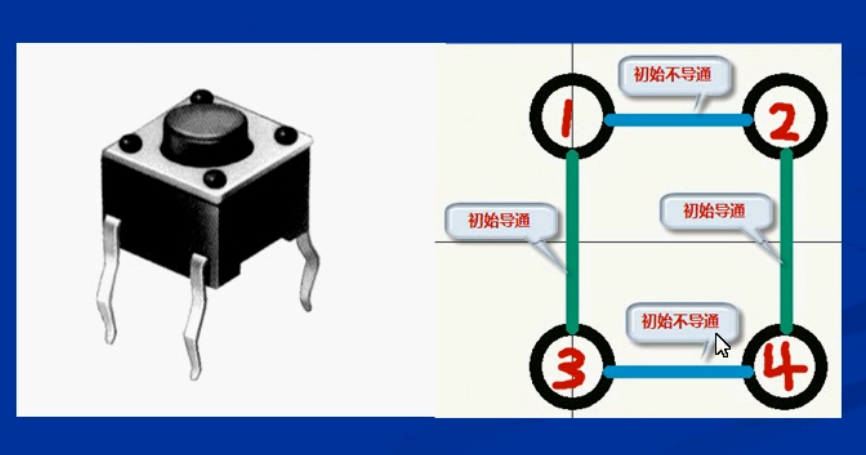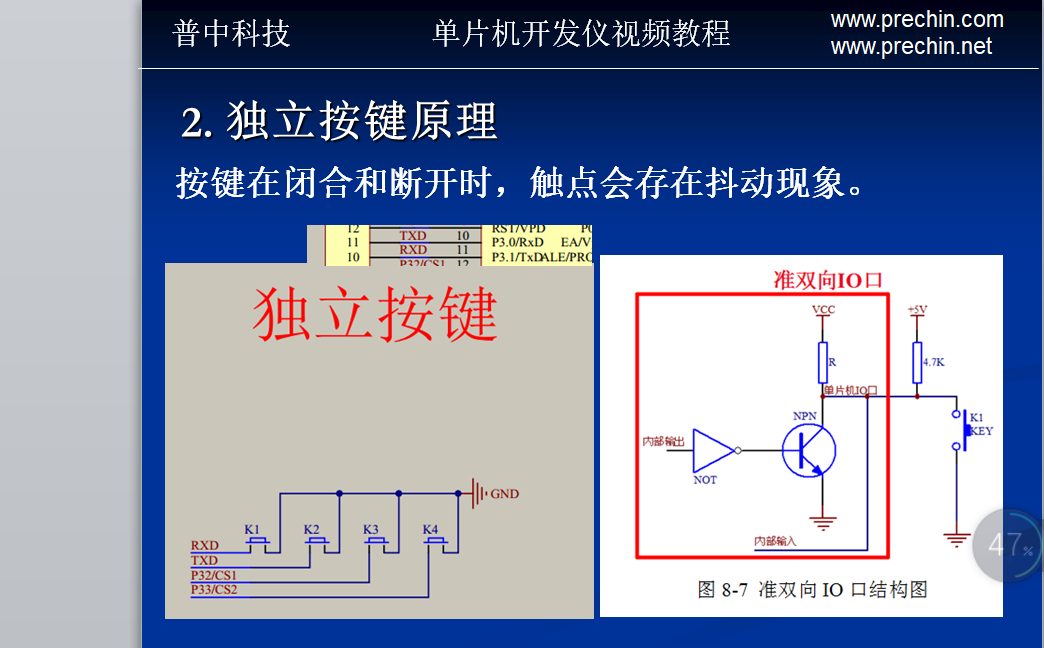## 51单片机之按键控制LED

2019-05-15 11:00:36 Steele_Jun 阅读数 1666
• ###### 0基础一个月学会51单片机-朱有鹏老师单片机系列视频课程第一季

26课时 486分钟 71人学习 朱有鹏
免费试看

• 按键k1连接在P3.1口; 按下按键，按键电平变为低电平；
• led1连接在P2.0口；led1低电平点亮；

``````#include "reg52.h"

typedef unsigned int u16;

sbit k1=P3^1;	 //定义P31口是k1
sbit led=P2^0;	 //定义P20口是led

void delay(u16 i)    // 10*i us
{
while(i--);
}

void key()
{
if(k1==0)		  //检测按键是否按下
{
delay(1500);   //抖动延时15ms
if(k1==0)	 //再次判断按键是否按下
{
led=~led;	  //按键按下，led状态取反
}
while(!k1);	 //检测按键是否松开，松开则退出；确保按键松开，不加这句控制不准确
}
}

void main()
{
while(1)
{
key();  //按键
}
}
``````

## 51单片机 4个独立按键控制LED灯 （protues仿真）（C语言版）

2019-08-19 21:48:16 qq_45420694 阅读数 7178
• ###### 0基础一个月学会51单片机-朱有鹏老师单片机系列视频课程第一季

26课时 486分钟 71人学习 朱有鹏
免费试看

# 51单片机 4个独立按键控制LED灯 仿真操作及代码展示 _ （protues仿真）_（C语言版）

## 二、有proteus及keil进行仿真效果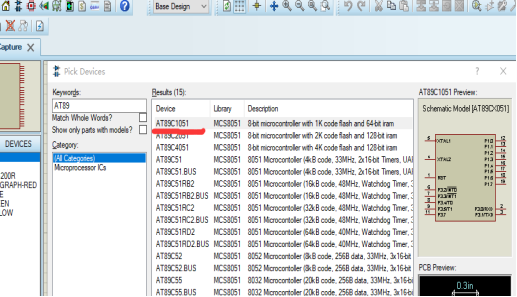第二步找到按键开关BUTTON及LED灯还有电阻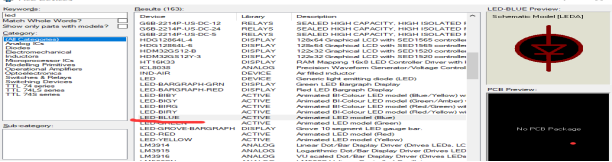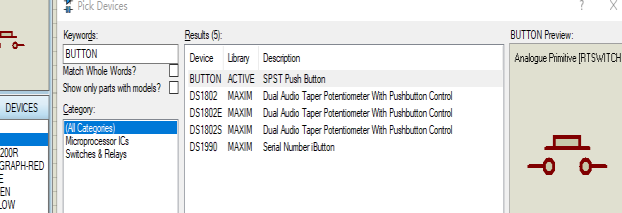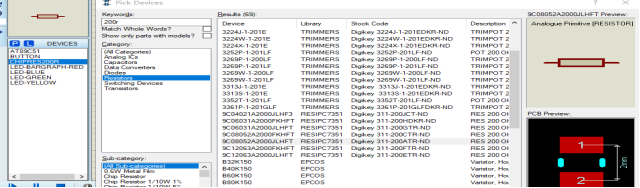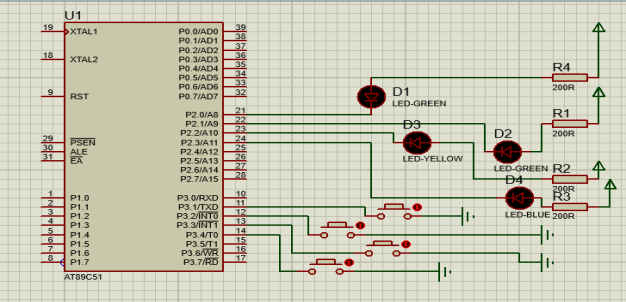## 三、打开keil4创建工程 代码及注释 调好波特率及添加文件相关工作 -----代码如下

#include<reg52.h>

unsigned int i;
sbit led1=P2^0; //定义第一个led灯
sbit led2=P2^1; //定义第二个led灯
sbit led3=P2^2; //定义第三个led灯
sbit led4=P2^3; //定义第四个led灯

sbit key1=P3^1; __ //定义第一个开关__
sbit key2=P3^2; //定义第二个开关
sbit key3=P3^3; //定义第三个开关
sbit key4=P3^4; //定义第四个开关

void delay() //延时函数 用于消抖
{
for(i=0;i<10;i++);
}
void key()
{
if(key10)
{
delay(); //延时 消抖
if(key1
0) //表示K1按下去
{
led1=0; // led1灯点亮
}
while(!key1); //表示_松开_按键key1
}
if(key20)
{
delay(); //延时 消抖
if(key2
0) //表示key2键被按下
{
led2=0; // led2灯点亮
}

``````  while(!key2);     //表示松开按键key2
}
if(k3==0)
{
delay();           //延时 消抖

if(key3==0)    //表示key3键被按下
{
led3=0;       // led3灯点亮
}
while(!key3);    //表示松开按键key3
}
if(key4==0)
{
delay();            //延时 消抖
if(key4==0)   //表示key4键被按下
{
led4=0;        // led4灯点亮
}
while(!key4);        //表示松开按键key4
}
``````

}

void main()
{
while(1)
{
key();
}
}